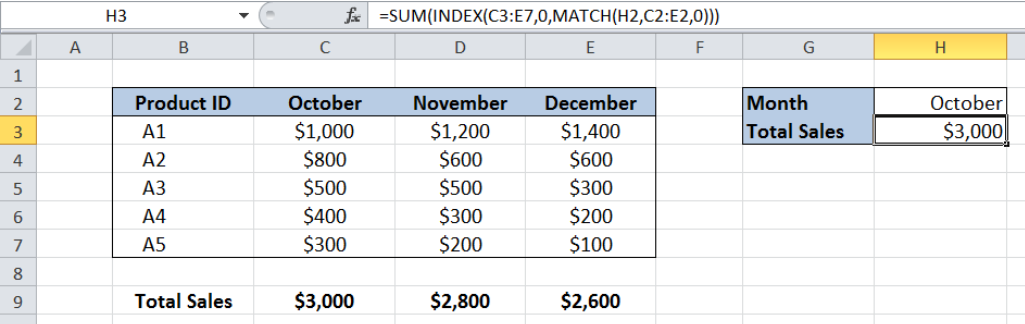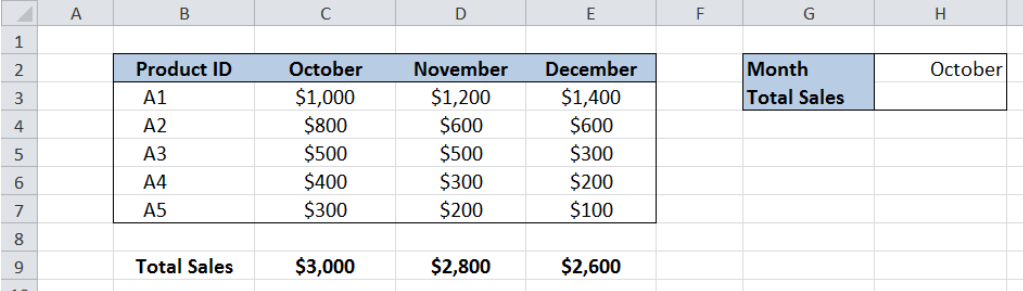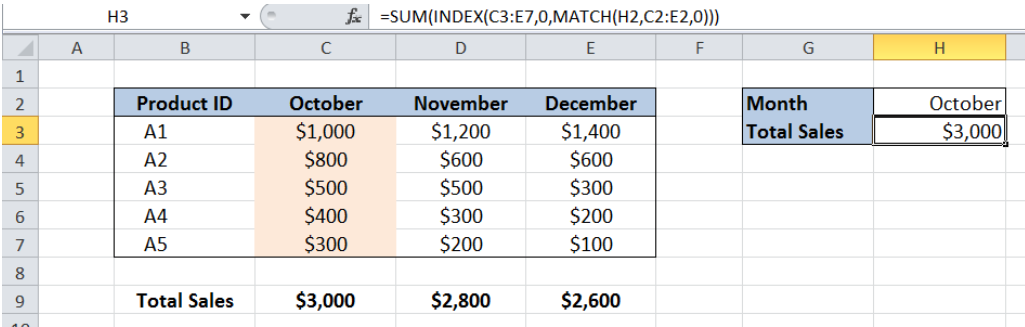Get instant live expert help with Excel or Google Sheets“My Excelchat expert helped me in less than 20 minutes, saving me what would have been 5 hours of work!”

#### Post your problem and you’ll get Expert help in seconds.

Your message must be at least 40 characters
Our professional Expert are available now. Your privacy is guaranteed.

# Lookup entire column

In Excel, we are able to lookup an entire column with the INDEX and MATCH functions.  The INDEX function returns a value as specified from within a range, while the MATCH function returns the position of a value in a range. This step by step tutorial will assist all levels of Excel users in looking up an entire column using INDEX and MATCH functions.Figure 1. Final result: Lookup entire column

Final formula: `=SUM(INDEX(C3:E7,0,MATCH(H2,C2:E2,0)))`

## Syntax of the INDEX function

`=INDEX(array, row_num, column_num)`

The parameters are:

• array – a range of cells where we want to retrieve some data
• row_num – the row in the array from which we want to retrieve data
• column_num – the column in the array from which we want to retrieve data

## Syntax of the MATCH function

`=MATCH(lookup_value, lookup_array, [match_type])`

The parameters are:

• lookup_value – a value which we want to find in the lookup_array
• lookup_array – the range of cells containing the value we want to match
• [match_type] optional; the type of match; if omitted, the default value is 1; We use 0 to find an exact match

## Setting up Our Data

Below table shows the sales of five products in the months of October (column C), November (column D) and December (column E).Figure 2. Sample data to lookup entire column

In cell H2, we specify the column whose values we want to lookup.  In cell H4, we want to determine the total sales for the month of October in order to show that we have retrieved all values in the entire column.

## Lookup an Entire Column

We want to determine the total sales for the month of October.Figure 3. Entering the formula to lookup entire column

To lookup entire column, we follow these steps:

Step 1.  Select cell H3.

Step 2.  Enter the formula: `=SUM(INDEX(C3:E7,0,MATCH(H2,C2:E2,0)))`

Step 3.  Press ENTER

• The array is the range C3:E7, which contains the data for Sales.
• We set the row number to zero “0” in order to lookup the entire column.
• The column number is determined by the MATCH function “MATCH(H2,C2:E2,0)”; October is in the first column of the lookup_array C2:E2 so column_num is 1.
• The final result in cell H3 is \$ 3,000, which is the total sales for the month of October.

Most of the time, the problem you will need to solve will be more complex than a simple application of a formula or function. If you want to save hours of research and frustration, try our live Excelchat service! Our Excel Experts are available 24/7 to answer any Excel question you may have. We guarantee a connection within 30 seconds and a customized solution within 20 minutes.

### Did this post not answer your question? Get a solution from connecting with the expert.Another blog reader asked this question today on Excelchat:
Solution examplesindex and match with duplicates. I need to use another column as a reference, so my return value has two match the value of two things for it to return
Solved by E. H. in 60 minsHello, I have a big spreadsheet in which I need to know how many patients came in each month based on provider. I am using the following formula but I still get the N/A error. =INDEX('No Show Appts Data'!D:E, MATCH(1, 'No Show Appts Data'!D:D='No Shows Data'!M2)*('No Shows Data'!E:E='No Shows Data'!N1),0)) No Show Appts Data is the name of the sheet where Column D is Month Year of date in question and Column E is the provider. No Shows Data is the sheet where I am making all the formula calculation where Column M is Month and Year and Column N is the provider in question and therefore M2 is the month in question and N1 the provider in question. How do I fix this error? Thanks
Solved by F. H. in 40 minsI need to find an INDEX function that will convert the Call Day (a number 1-7) to the actual weekday found in row 1 of the DayofWeek named range. I don't know if I'm supposed to use the MATCH function as well or not.
Solved by D. D. in 11 minsI have two columns with names and a third one with email addresses. For every name that matches, I need to copy the email address to an empty column next to the matching name. .
Solved by E. U. in 15 minshelp with INDEX(IndexArea,MATCH(\$M\$5,MatchRows,0),MATCH(\$M\$6,MatchColumns,0))
Solved by Z. Y. in 12 mins## Subscribe to Excelchat.coAnother blog reader asked this question today on Excelchat: